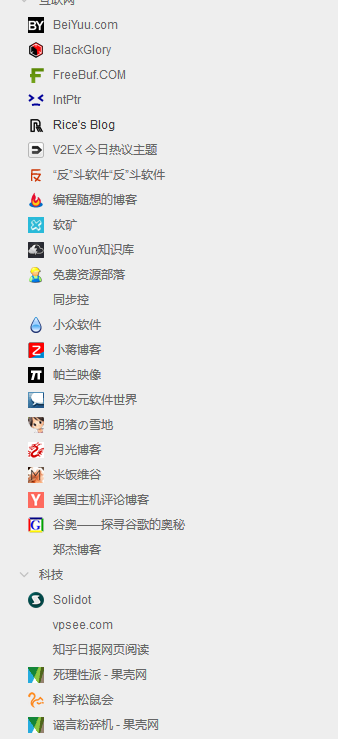## 分享我的 Star 文章 rss 订阅

http://best33.com/usr/stared_feed.php/

pentadactyl 是一个以类 vim 方式操作 Firefox 的插件。

yum install mercurial zip make # 安装hg，zip，gmake等依赖
hg clone http://dactyl.googlecode.com/hg/ dactyl # 将 dactyl 的源码克隆到本地
cd dactyl/


# 更新：Firefox 35 可用的版本已经传到了百度网盘。

/

## 使用 InstantClick 时 piwik 、 MathJax 的配置

InstantClick 是一个利用 hover 和 click 的时间差，预加载网页的黑科技。原理主要是在 hover 的时候预读网页，然后点击的时候就替换整个页面，让你的网站看起来整个都 PJAX 过~很酷炫。

InstantClick.on('change', function() {
//piwik
_paq.push(['setDocumentTitle', document.title]);
_paq.push(['trackPageView']);
//mathjax
MathJax.Hub.Queue(["Typeset",MathJax.Hub]);
});
InstantClick.init();


Enjoy ~

/

## supervisord 管理多进程程序如 pyspider 时的合适配置

If true, the flag causes supervisor to send the stop signal to the whole process group and implies killasgroup is true. This is useful for programs, such as Flask in debug mode, that do not propagate stop signals to their children, leaving them orphaned.

[program:pyspider]
command=/home/oott123/pyspider/run.py
autorestart=true
startsecs=2
user=oott123
directory=/home/oott123/pyspider/
redirect_stderr=true
stopasgroup=true
environment=WEBUI_HOST="127.0.0.1",WEBUI_PORT="5033"


/

## 如何安装 docker

docker 在 CentOS 7 下已经收录到了 extras 包中，因此我们只需要执行：yum install docker 即可安装 docker。

[root@localhost data]# docker info
Containers: 0
Images: 0
Storage Driver: devicemapper
Pool Name: docker-253:0-68161190-pool
Data file: /var/lib/docker/devicemapper/devicemapper/data
Data Space Used: 293.0 Mb
Data Space Total: 102400.0 Mb
Execution Driver: native-0.2
Kernel Version: 3.10.0-123.el7.x86_64


## 如何使用国内的 docker 镜像源

docker pull dl.dockerpool.com:5000/centos:centos7


docker tag dl.dockerpool.com:5000/centos:centos7 centos:centos7


## 如何清理 docker 容器

Log() { echo $(date +"[%Y-%m-%d %H:%M:%S]")$@; }
Log ====每日备份脚本开始运行====
REMOTE_DIR=$(date +"%Y%m")/$(date +"%Y%m%d") # 远端上传路径
WORKING_DIR="/root/auto-scripts/backup_daily" # 工作路径
CONFIG_DIR=$WORKING_DIR/list.d #配置文件路径 BACKUP_DIR=$WORKING_DIR/backups #本地备份路径
CURRENT_DIR=$BACKUP_DIR/$(date +"%Y%m%d")
OLD_DIR=$BACKUP_DIR/$(date -d -3day +"%Y%m%d")
#准备删除老文件
Log 删除旧备份文件夹：$OLD_DIR rm -rf$OLD_DIR
#创建新目录
mkdir -p $CURRENT_DIR #遍历配置目录，进行备份 for i in$(ls $CONFIG_DIR) do zipExclude="*/cache/* */tmp/* */temp/* */.npm/* */data/threadcache/*" .$CONFIG_DIR/$i Log 备份$backupDir为$i…… backupFile=$i.zip
zip -r -P $zipPassword$CURRENT_DIR/$backupFile$backupDir -x $zipExclude Log 上传备份……$UPLOADER_BIN upload $CURRENT_DIR/$backupFile $REMOTE_DIR/$backupFile
done
Log 备份完成！


/

# 模型表达

$$g(z) = {1 \over {1 + {e^{ – z}}}}$$

$${h_\theta }(x) = {1 \over {1 + {e^{ – {\theta ^T}x}}}}$$

# 决策边界（Decision boundary）

（啊突然发现真的有点不得不配图了……我还要再坚持一下！）

# 成本函数

$$J(\theta ) = – {1 \over m}[\sum\limits_{i = 1}^m {{y^{(i)}}\log {h_\theta }({x^{(i)}}) + (1 – {y^{(i)}})\log (1 – {h_\theta }({x^{(i)}}))]}$$

$${\partial \over {\partial {\theta _j}}}J(\theta ) = {1 \over m}\sum\limits_{i = 1}^m {({h_\theta }({x^{(i)}}) - {y^{(i)}})x_j^{(i)}}$$

• BFGS
• L-BFGS

/

# 模型表达

$${{\rm{h}}_\theta }(x) = {\theta _0} + {\theta _1}{x_1} + {\theta _2}{x_2} + … + {\theta _n}{x_n}$$

——其实有 $$x_0$$ 的，只是它等于1，于是就没有 $$x_0$$ 了。

$${{\rm{h}}_\theta }(x) = {\theta ^T}x$$

# 多变量的梯度下降算法

## 成本函数

$$J(\theta ) = {1 \over {2m}}\sum\limits_{i = 1}^m {{{({h_\theta }({x^{(i)}}) – {y^{(i)}})}^2}}$$

# 特征缩放（Feature Scaling）

$${{x – \mu } \over s}$$

# 学习速率（Learning rate， $$\alpha$$）

$$\theta_{j}:=\theta_{j}-\alpha\frac{\partial}{\partial\theta_{j}}J(\theta_{0},\theta_{1})$$

# 一般等式

$$\theta = {({X^T}X)^{ – 1}}{X^T}{\rm{y}}$$

/

## 根据天气和时间动态更换的博客背景图

var t = Math.floor(((new Date()).getHours() + 6) / 5),
f = 'bright' + t;
if (w.indexOf('雨')>=0 || w.indexOf('雪') >= 0) {
f = 'rainy';
} else if (w.indexOf('云')>=0 || w.indexOf('阴') >= 0) {
f = 'cloudy' + t;
}
if (document.body.clientWidth > 600) {
document.body.style.backgroundImage = 'url("' + f + '.jpg")';
}


/

# 模型表达

• $$m$$ 代表训练数据集的大小（即有多少个训练样本）；
• $$x$$ 代表模型的输入（即模型的输入特征数量）；
• $$y$$ 代表模型的输出（即最终预测的结果）；
• 我们用上标 $${^{(i)}}$$代表数据集中的第 i 组数据，如 $${x^{(i)}}$$ 代表数据集中第二个输出；
• $$h$$ 则是我们用于预测的函数，具体表述如下：
将学习算法通过训练数据集训练之后得出函数 $$h$$ 中的不确定参数项，此时 $$h$$ 为一确定的函数；
将任意的输入 $$x$$ 代入 $$h$$ 中，即可得到对应的输出 $$y$$。
在单变量线性回归中，我们使用的 $${h_\theta}(x)$$$${h_\theta }(x) = {\theta _0} + {\theta _1}x$$

# 梯度下降法

repeat until convergence {
$$\theta_{j}:=\theta_{j}-\alpha\frac{\partial}{\partial\theta_{j}}J(\theta_{0},\theta_{1})$$ (for j=0 and j=1)
}

** 值得注意的是，此处的 $$\theta$$ 的更新是同步的，亦即计算$$\theta_2$$时使用的$$\theta_1$$是本次更新前的值。**

$${\theta _0}: = {\theta _0} - \alpha {1 \over m}\sum\limits_{i = 1}^m {({h_\theta }({x^{(i)}}) - {y^{(i)}})}$$$ $${\theta _1}: = {\theta _1} - \alpha {1 \over m}\sum\limits_{i = 1}^m {({h_\theta }({x^{(i)}}) - {y^{(i)}})} \cdot {x^{(i)}}$$$

/